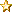April 08, 2020, 12:55:29 pm
 News: Want to see a specific Tutorial? ASK!# Cartesian to Polar coordinates and vice-versa

 Pages:Author Topic: Cartesian to Polar coordinates and vice-versa  (Read 1358 times)
Quark
Post DemosPosts: 14« on: June 26, 2011, 06:00:37 pm »

Hi all,

Sometime in the past I did some research on this, then forgot all about it, and recently went back and did the same thing.  The info on the web is extensive, but often confusing to a non-math person such as myself.

When one is moving things around on either the text screen or a graphics one, basically it is a Cartesian (or rectangular) grid with the horizontal axis being x and the vertical one as y.  The position of a point is given as x,y.  This is exceedingly cool and a lot can be done with it.  However, if you want to think about moving something on the grid, it is actually awkward to do if you want to do it in a "natural" way.  Meaning if you imagine a dragon (or whatever) moving, the x,y business is tiresome.  That is where the Polar coordinate system is handy.

With the Polar system you can imagine moving your dragon at, say, thirty degrees heading to a distance of 10, or again you want your dragon to fire molten lava blobs at an enemy, and you want to aim him 190 degrees with distance 25.  Easy with polar, hard with Cartesian.

So, the need is to have two subroutines that convert back and forth from one coordinate system to the other.  When, for example you want to know where that dragon would end up with a 45 degree turn and a move of 12, you would translate that to Cartesian coordinates and add the results to the current position of the dragon.

The program below shows the behavior of the routines.  Hope you find this useful -- it's especially good for gaming.

--Quark

Code:
DEFDBL A-Z
SCREEN _NEWIMAGE(800, 600, 256)
rows = INT(_WIDTH / 8): cols = INT(_HEIGHT / 16)
COLOR 10

r = 1
FOR theta = 0 TO 360 STEP 30
PolarToCartesian r, theta, x, y
PRINT USING "With dist of 1 and angle ###.## the Cartesian coords are ###.## and ###.##"; theta, x; y
NEXT
PRINT

FOR x = 2 TO 8 STEP 2: FOR y = 2 TO 8 STEP 2 ' Cartesian (grid) locations
CartesianToPolar x, y, r, theta
PRINT USING "With x and y of ###.## and ###.## Polar coords are: ###.## and ###.##"; x; y; r; theta
NEXT: NEXT
END
'-----------------------------------------------------------------------------
SUB PolarToCartesian (r AS DOUBLE, theta AS DOUBLE, x AS DOUBLE, y AS DOUBLE)
'r=distance, theta = angle in degrees, x and y will hold the result
x = r * COS(theta * ((4 * ATN(1)) / 180)): y = r * SIN(theta * ((4 * ATN(1)) / 180))
END SUB
'-----------------------------------------------------------------------------
SUB CartesianToPolar (x AS DOUBLE, y AS DOUBLE, r AS DOUBLE, theta AS DOUBLE)
' x and y are the Cartesian (rectangular) coordinates, r and theta hold the result
r = SQR(x * x + y * y): theta = ATN(y / x) / ((4 * ATN(1)) / 180) 'to degrees
END SUB
'-----------------------------------------------------------------------------

 Report SpamLogged

 Pages: